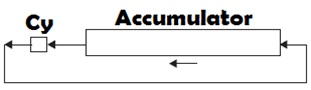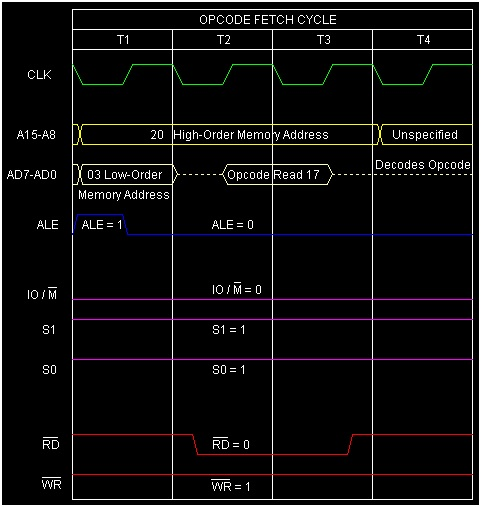# Instruction type RAL in 8085 Microprocessor

In 8085 Instruction set, there is another mnemonic RAL,which stands or Rotate Accumulator Left and also involving Cy flag in rotation. It rotates the Accumulator contents to the left by 1-bit position. The following Fig. is depicting the execution logic of the instruction.From the Fig. we can see that the due to left rotation the bit which is coming out from the most significant place will be copied to the Cy flag bit. And the previous Cy bit will be moved to least significant bit place of the Accumulator. Thus it is a 9-bit rotation of Accumulator and Cy contents. Only Cy flag is affected by this instruction execution.

Mnemonics, OperandOpcode(in HEX)Bytes
RAL171

The instruction can be used for multiple purposes.

• To find the most significant bit of the Accumulator, performing rotate left.

• To perform multiplication by 2. We know that if we multiply a number by 2n then the number will have n-bit left rotation.

• As during rotation, an empty space will get created. So to put a new bit at that least significant space we can use this mnemonic as well.

Let us consider the following examples for the better understanding.

## Example 1

35H ---> 0011 0101
0 0110 1010 ---> 6AH (Prev. Cy bit as the Last bit)

BeforeAfter
(A)
35H6AH
(Cy)
00

200317RALRotateAccumulator Left

Note that Accumulator value has been doubled compared with the previous value.

## Example 2

35H ---> 0011 0101
0 0110 1011 ---> 6BH(Prev. Cy bit as the Last bit)

BeforeAfter
(A)
35H6BH
(Cy)
10